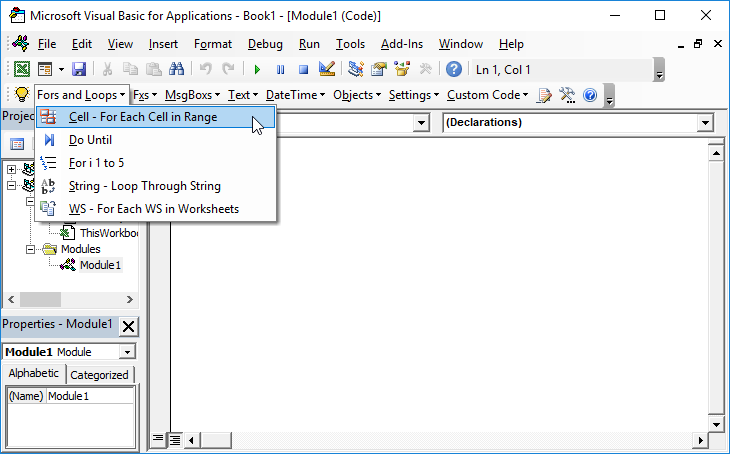# VBA – Sum by Color

The following function calculates the total value of all the cells in a particular range that are a particular color:

Unfortunately, there is no SUMIF Function to sum based on cell color. If you want to sum by color, you will need to create a function within VBA.
To use this code: Open the Visual Basic Editor (Alt + F11), Insert a new module (Insert > Module) and copy & paste the desired code into the module.

## Function to Sum by Color

``````
Function Color_By_Numbers(Color_Range As Range, Color_Index As Integer) As Double

' Dim Color_By_Numbers  As Double
Dim Cell

'Will look at cells that are in the range and if
'the color interior property matches the cell color required
'then it will sum

'Loop Through range

For Each Cell In Color_Range

If (Cell.Interior.ColorIndex = Color_Index) Then
Color_By_Numbers = Color_By_Numbers + Cell.Value
End If

Next Cell

End Function
``````

This is in effect “sum by color” – so if you know Excel’s 56 color palatte and you know for example that color 4 is light green then the following call:

Color_By_Numbers(“A1:P20”,4)

will sum the values for all the cells in the range A1:P20 that are light green in color.

To make using the function easier, the following subroutine will work out the total value for each of excel’s 56 colors. It also gives the entire palatte so that it is easy to see the index number for each color.

The subroutine is invoked on sheet 1 and looks at the range

``````
Private Sub CommandButton1_Click()

'Will look at each color and produce summary table of values
'on sheet 1 in cell A1 and downwards

Dim Current_Color_Number As Integer
Dim Color_Total As Double

For Current_Color_Number = 1 To 56

Color_Total = Color_By_Numbers(Sheets("Sheet2").Range("a11:aa64"), Current_Color_Number)

Worksheets("Sheet1").Range("A1").Offset(Current_Color_Number, 0) = Current_Color_Number
Worksheets("Sheet1").Range("A1").Offset(Current_Color_Number, 0).Interior.ColorIndex = Current_Color_Number

If Color_Total  0# Then
Worksheets("Sheet1").Range("a1").Offset(Current_Color_Number, 1).Value = Color_Total
End If

Next Current_Color_Number

End Sub
``````